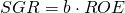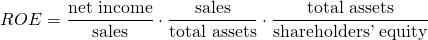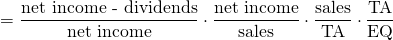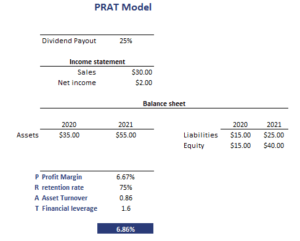# PRAT Model

The PRAT model is a method to calculate the sustainable growth rate using the DuPont formula. The sustainable growth rate is the rate at which earnings (and dividends) can continue to grow indefinitely, assuming that the firm’s debt-to-equity ratio does not change and it does not raise additional equity.

We discuss the relationship between the sustainable growth rate and the DuPont formula, discuss the resulting PRAT model formula, and finally implement an example in Excel. The spreadsheet is included at the bottom of the page.

## PRAT model formula

First, let’s estimate the sustainable growth rate using the DuPont formula. To do that, we first start from the following relationship between return on equity (ROE) and the sustainable growth rate g.Next, we know that ROE can be decomposed using the DuPont formula as followsfinally, we can combine both formulas. Thus, g can be calculated asThis is the PRAT formula, which shows that the SGR is a function of the profit margin (P), the retention rate (R), the asset turnover (A), and financial leverage (T).

## PRAT model interpretation

The PRAT model indicates that a firm’s growth rate depends on two kinds of factors. On the one hand, there are the financing decisions (leverage and earnings retention). On the other hand, there’s performance (profit margin and asset turnover).

If the actual growth forecasted by an analyst is greater than the SGR, then this implies that the company will have to issue more equity unless the firm increases its leverage, profit margin, total asset turnover, or retention rate.

## PRAT example

Let’s apply the model to some actual data. The figure below shows how we can combine data on the different components of the model to estimate the sustainable growth rate.## Summary

We discussed the PRAT model, a method to calculate the sustainable growth rate. The advantage of the PRAT model is that it provides a lot of insight on the drivers of the sustainable growth rate.

### PRAT

Want to have an implementation in Excel? Download the Excel file: PRAT